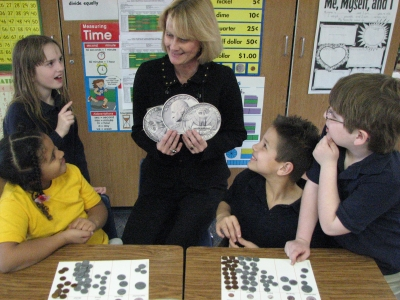Recognizing number patterns is also an important problem-solving skill. If you see a pattern when you look systematically at specific examples, you can use that pattern to generalize what you see into a broader solution to a problem.

The youngest children begin simply by counting. They count by 1s, then by 2s, 5s, and 10s. These patterns give students a natural strategy to understand addition and multiplication. When considering a number pattern such as 2, 4, 6..., a young student will ask herself, By what number can I count (add) to get to the next number in the pattern and the next and the next?

As the student gets older, her knowledge of patterns advances from sums to products. When asked for the 50th number in the pattern, she will know to multiply 2 times 50. High school students can start to understand functions, such as f(x) = 2x + 2, where x is the numerical sequence 0, 1, 2, 3,…. They begin with simple in-out machines and gradually adapt their understanding to the abstractions of algebra.

### Standards

NCTM Standard 2 (1998) sets the purpose of patterns, functions, and algebra in mathematics education at all grade levels.

Mathematics instructional programs should include attention to patterns, functions, symbols, and models so that all students

• understand various types of patterns and functional relationships;
• use symbolic forms to represent and analyze mathematical situations and structures;
• use mathematical models and analyze change in both real and abstract contexts.

Han-5 System reinforces the conceptual understanding of mathematics. Students learn different nine patterns to understand and recall multiples.  Then they can solve many kinds of algorithms.
Here is a picture that  portrays a million words!  These four students learned Han-5 one month ago.  Now they can apply  the "Froggie Four" hand pattern with money to understand how to solve problems with quarters. Why... because quarters are multiples of four !
Relationships are a cool way to understand functions and operations with math.